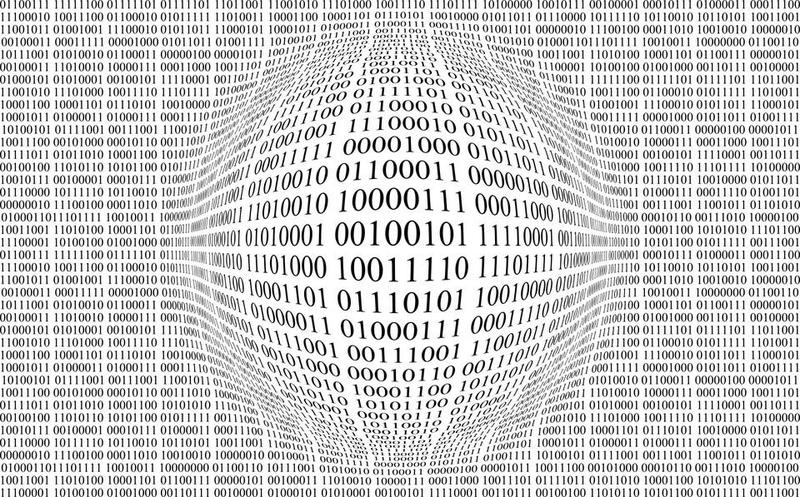Number Bases: How binary, hex, and other number systems workwritten by
brian### Introduction

Because of how computers work at a base electronic level, it's common to find code that makes use of numbers that are written differently than the decimal system that most people are used to. In this module, we'll first dissect the base-10 (decimal) system that we use every day, then apply what we've learned to change to other number systems, including binary and hexadecimal. Finally, we'll briefly talk about why almost all modern computers use a binary number system to perform calculations.

### Objectives

• Understand number bases: base-10, base-16, base-2
• Convert numbers between bases
• Have a general understanding of why we use different bases